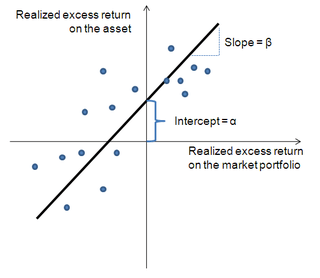# Security characteristic lineSecurity characteristic line

Positive abnormal return (α): Above-average returns that cannot be explained as compensation for added risk

Negative abnormal returns (α): Below-average returns that cannot be explained by below-market risk

Security characteristic line (SCL) is a regression line, plotting performance of a particular security or portfolio against that of the market portfolio at every point in time. The SCL is plotted on a graph where the Y-axis is the excess return on a security over the risk-free return and the X-axis is the excess return of the market in general. The slope of the SCL is the security's beta, and the intercept is its alpha.

## Formulawhere:

αi is called the asset's alpha (abnormal return)
βi(RM,t – Rf) is a nondiversifiable or systematic risk
εi,t is the non-systematic or diversifiable, non-market or idiosyncratic risk
RM,t is a market risk
Rf is a risk-free rate